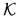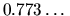Next: Deborah S. Franzblau - Up: Discrete Geometry / Géométrie Previous: Michel Deza and Mikhail

## Ferenc Fodor - Large polygons in convex sets and polygons with large perimeter

 FERENC FODOR, Department of Mathematics, Auburn University, Auburn, Alabama  36849-5310, USA Large polygons in convex sets and polygons with large perimeter

In this talk we investigate the problem that how large convex n-gons can be inscribed in a convex discin its Minkowski metric. We show that in any convex disc we can always inscribe a triangle of minimal side length 2/3, and in a centrally symmetric disc a triangle with sides not shorter than. We also give a short construction for an inscribed triangle whose sides are at least 3/4in the Minkowski metric induced by.

We also consider the question of finding the maximum perimeter pnof a convex n-gon of unit diameter. We prove that the diameter graph of such an extremal polygon must be connected. Therefore if n=2kno regular polygon is extremal. We shall also present applications of these facts in connection with finite circle packing problems and diameter minimalization of finite point sets with mutual distances at least one.

Some of the above results are joint works with A. Bezdek and I. Talata.Next: Deborah S. Franzblau - Up: Discrete Geometry / Géométrie Previous: Michel Deza and Mikhail